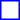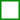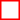ArcGIS REST Services Directory Login | Get Token

## Legend (precip/wpc_prob_winter_precip)

Day 1 probability of at least 4 inches of snow (1)SLGT: AT LEAST 10% PROBMDT: AT LEAST 40% PROBHIGH: AT LEAST 70% PROB
Day 1 probability of at least 8 inches of snow (2)SLGT: AT LEAST 10% PROBMDT: AT LEAST 40% PROBHIGH: AT LEAST 70% PROB
Day 1 probability of at least 12 inches of snow (3)SLGT: AT LEAST 10% PROBMDT: AT LEAST 40% PROBHIGH: AT LEAST 70% PROB
Day 1 probability of icing >0.25 inches (4)SLGT: AT LEAST 10% PROBMDT: AT LEAST 40% PROBHIGH: AT LEAST 70% PROB
Day 2 probability of at least 4 inches of snow (6)SLGT: AT LEAST 10% PROBMDT: AT LEAST 40% PROBHIGH: AT LEAST 70% PROB
Day 2 probability of at least 8 inches of snow (7)SLGT: AT LEAST 10% PROBMDT: AT LEAST 40% PROBHIGH: AT LEAST 70% PROB
Day 2 probability of at least 12 inches of snow (8)SLGT: AT LEAST 10% PROBMDT: AT LEAST 40% PROBHIGH: AT LEAST 70% PROB
Day 2 probability of icing >0.25 inches (9)SLGT: AT LEAST 10% PROBMDT: AT LEAST 40% PROBHIGH: AT LEAST 70% PROB
Day 3 probability of at least 4 inches of snow (11)SLGT: AT LEAST 10% PROBMDT: AT LEAST 40% PROBHIGH: AT LEAST 70% PROB
Day 3 probability of at least 8 inches of snow (12)SLGT: AT LEAST 10% PROBMDT: AT LEAST 40% PROBHIGH: AT LEAST 70% PROB
Day 3 probability of at least 12 inches of snow (13)SLGT: AT LEAST 10% PROBMDT: AT LEAST 40% PROBHIGH: AT LEAST 70% PROB
Day 3 probability of icing >0.25 inches (14)SLGT: AT LEAST 10% PROBMDT: AT LEAST 40% PROBHIGH: AT LEAST 70% PROB